Test: Time Varying EMT Fields - 1

# Test: Time Varying EMT Fields - 1 - Electrical Engineering (EE)

Test Description

## 10 Questions MCQ Test Topicwise Question Bank for Electrical Engineering - Test: Time Varying EMT Fields - 1

Test: Time Varying EMT Fields - 1 for Electrical Engineering (EE) 2023 is part of Topicwise Question Bank for Electrical Engineering preparation. The Test: Time Varying EMT Fields - 1 questions and answers have been prepared according to the Electrical Engineering (EE) exam syllabus.The Test: Time Varying EMT Fields - 1 MCQs are made for Electrical Engineering (EE) 2023 Exam. Find important definitions, questions, notes, meanings, examples, exercises, MCQs and online tests for Test: Time Varying EMT Fields - 1 below.
Solutions of Test: Time Varying EMT Fields - 1 questions in English are available as part of our Topicwise Question Bank for Electrical Engineering for Electrical Engineering (EE) & Test: Time Varying EMT Fields - 1 solutions in Hindi for Topicwise Question Bank for Electrical Engineering course. Download more important topics, notes, lectures and mock test series for Electrical Engineering (EE) Exam by signing up for free. Attempt Test: Time Varying EMT Fields - 1 | 10 questions in 30 minutes | Mock test for Electrical Engineering (EE) preparation | Free important questions MCQ to study Topicwise Question Bank for Electrical Engineering for Electrical Engineering (EE) Exam | Download free PDF with solutions
 1 Crore+ students have signed up on EduRev. Have you?
Test: Time Varying EMT Fields - 1 - Question 1

### If the magnetic field vector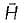has only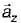component given by Hz = 3x cosβ+ 6y sinγ and if the field is invariant with time, what is the expression for current density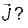Detailed Solution for Test: Time Varying EMT Fields - 1 - Question 1

Since,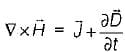and as the field is invariant, therefore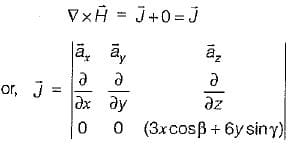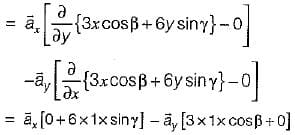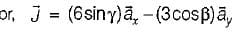Test: Time Varying EMT Fields - 1 - Question 2

### The conduction current in a straight copper wire of cross-sectional area A = 1.5 x 10-5 m2 is I = 2 A. If the constitutive parameters of copper are μ = μ0 = 4π x 10-7 H/m,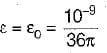F/m and σ = 5.8 x 107 mho/m, then the displacement current Id in the wire at a frequency of f = 1 GHz would be

Detailed Solution for Test: Time Varying EMT Fields - 1 - Question 2

Let the copper wire lies along the z-axis of the cylindrical coordinate system. The magnetic field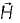is then directed in azimuthal direction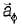and electric field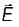is directed in the z-direction.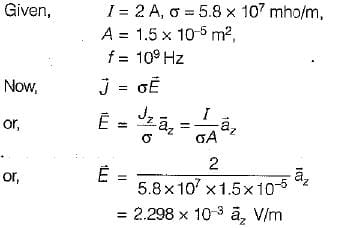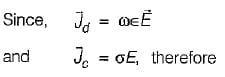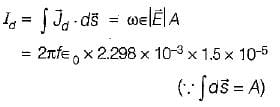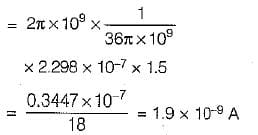or, Id = 1.9 nA

Test: Time Varying EMT Fields - 1 - Question 3

### A rectangular loop of length a = 1 meter and width b = 80 cm is placed in a uniform magnetic field. What is the maximum value of induced emf if the magnetic flux density B = 0.1 Wb/m2 is constant and the loop rotates about the x-axis with a frequency of 50 Hz?

Detailed Solution for Test: Time Varying EMT Fields - 1 - Question 3

The induced emf in uniform magnetic field is given by
v = ω(ab) B sin ωt
or, v = ωAB sin ωt
(where, A = ab = Area of rectangular loop)
or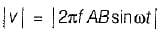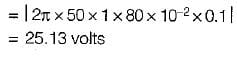Test: Time Varying EMT Fields - 1 - Question 4

A straight conducting wire of length 50 cm is moved in a direction at right angles to its length in a region in air permitted by a uniform flux density B of magnitude B = 1 Wb/m2. If the magnetic flux density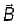is perpendicular to both the direction of the motion and the length of the wire and the magnitude of the velocity of motion \/ = 10 m/s, the induced voltage in the conductor would be

Detailed Solution for Test: Time Varying EMT Fields - 1 - Question 4

Induced emf in the conductor is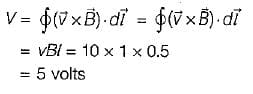Test: Time Varying EMT Fields - 1 - Question 5

A Faraday disc of 10 cm radius rotates at 5000 rpm in a uniform magnetic field of 250 mWb/m2, the field being normal to the plane of the disc. What is the emf between rpm and axis assuming the diameter of the axis very small?

Detailed Solution for Test: Time Varying EMT Fields - 1 - Question 5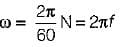where, N = Number of revolutions/min
∴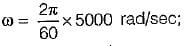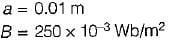The emf induced is,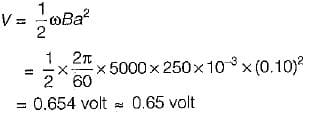Test: Time Varying EMT Fields - 1 - Question 6

What is the emf developed about the path r = 0.5, z = 0 and at t = 0, if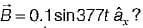Detailed Solution for Test: Time Varying EMT Fields - 1 - Question 6

Given B = 0.1 Wb/m2
r = 0.5 m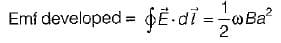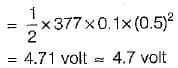Test: Time Varying EMT Fields - 1 - Question 7

A square loop of wire 25 cm has a voltmeter (of infinite impedance) connected in series with one side. The plane of the loop is perpendicular to the magnetic field and the frequency is 10 MHz. If the maximum intensity is 1 Amp/m, then the voltage indicated by the meter when the loop is placed in the alternating field would be

Detailed Solution for Test: Time Varying EMT Fields - 1 - Question 7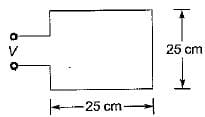Given,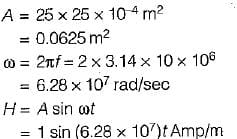The required induced voltage is given by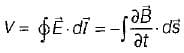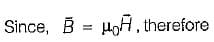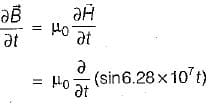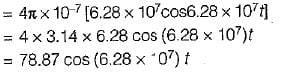Hence, the maximum induced voltage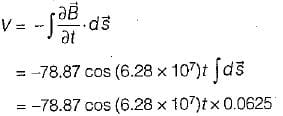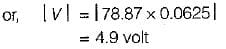Test: Time Varying EMT Fields - 1 - Question 8

Assertion (A): The total e.m.f. induced in a circuit is equal to the time rate of decrease of the total magnetic flux linking the circuit.
Reason (R): Changing magnetic field will induce on electric field.

Detailed Solution for Test: Time Varying EMT Fields - 1 - Question 8

Both assertion and reason are individually correct statements.
Since induced emf,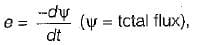thus due to negative sign, the total emf induced is equal to the time rate of decrease of the total magnetic flux linking the circuit. (The negative sign supports the Lenz’s law).

Test: Time Varying EMT Fields - 1 - Question 9

Assertion (A): Motional induction or flux cutting law gives the e.m.f. induced in a moving conductor w.r.t observer in a magnetic field.
Reason (R): The motional emf equation depends on the velocity of the conductor and its position.

Detailed Solution for Test: Time Varying EMT Fields - 1 - Question 9
• Assertion (A) is a correct statement since the motional induction or flux cutting law are given by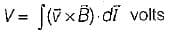or, V = vBl volts
• Motional emf equation depends only on the velocity of the conductor and not its position. Thus, reason (R) is not a correct statement,
Test: Time Varying EMT Fields - 1 - Question 10

In a material for which conductivity σ = 5 Siemen/m and ∈r = 1, the electric field intensity is E = 250 sin 1010 t V/m. What is the frequency at which the displacement and conduction current densities will be equal?

Detailed Solution for Test: Time Varying EMT Fields - 1 - Question 10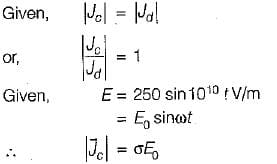= Conduction current density
and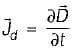= Displacement current density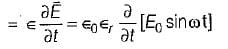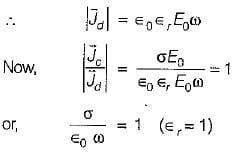or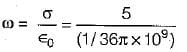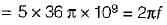or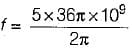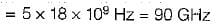## Topicwise Question Bank for Electrical Engineering

211 tests
Information about Test: Time Varying EMT Fields - 1 Page
In this test you can find the Exam questions for Test: Time Varying EMT Fields - 1 solved & explained in the simplest way possible. Besides giving Questions and answers for Test: Time Varying EMT Fields - 1, EduRev gives you an ample number of Online tests for practice

211 tests